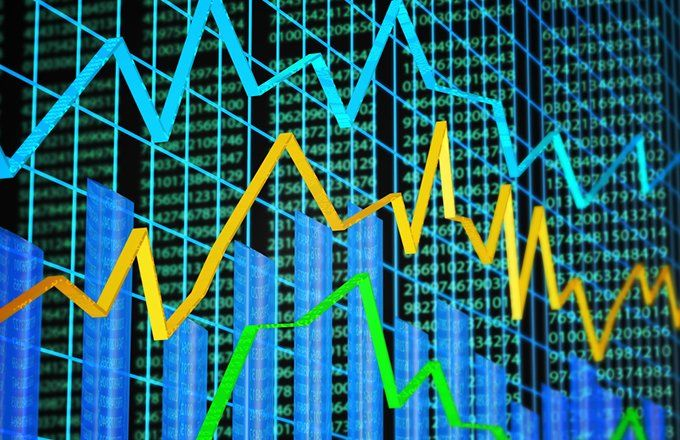The economic order quantity (EOQ) model is used in inventory management by calculating the number of units a company should add to its inventory with each batch order to reduce the total costs of its inventory. The costs of its inventory include holding and setup costs.

The EOQ model seeks to ensure that the right amount of inventory is ordered per batch so a company does not have to make orders too frequently and there is not an excess of inventory sitting on hand. It assumes that there is a trade-off between inventory holding costs and inventory setup costs, and total inventory costs are minimized when both setup costs and holding costs are minimized.

### The Formula for Economic Order Quantity

﻿EOQ=2×S×DHwhere:S=Setup costs (per order, generally including shipping and handling)D=Demand rate (quantity sold per year)\begin{aligned} &EOQ= \sqrt{\frac{2\times S\times D}{H}}\\ &\textbf{where:}\\ &S = \text{Setup costs (per order, generally including shipping and handling)}\\ &D = \text{Demand rate (quantity sold per year)}\\ &H = \text{Holding costs (per year, per unit)} \end{aligned}

### How to Calculate the Economic Order Quantity

To calculate the economic order quantity for inventory you must know the setup costs, demand rate, and holding costs.

Setup costs refer to all of the costs associated with actually ordering the inventory, such as the costs of packaging, delivery, shipping, and handling.

Demand rate is the amount of inventory a company sells each year.

Holding costs refer to all the costs associated with holding additional inventory on hand. Those costs include warehousing and logistical costs, insurance costs, material handling costs, inventory write-offs and depreciation.

Ordering a large amount of inventory increases a company’s holding cost while ordering smaller amounts of inventory more frequently increases a company’s setup costs. The economic order quantity model finds the quantity that minimizes both types of costs.

### Example of How EOQ Works

EOQ considers the timing of reordering, the cost incurred to place an order, and costs to store merchandise. If the company is constantly placing small orders to maintain a specific inventory level, the ordering costs are higher, along with the need for additional storage space.

For example, consider a retail clothing shop that carries a line of men’s shirts. The shop sells 1,000 shirts each year. It costs the company $5 per year to hold a single shirt in inventory, and the fixed cost to place an order is$2.

The EOQ formula is the square root of (2 x 1,000 shirts x $2 order cost) / ($5 holding cost) or 28.3 with rounding. The ideal order size to minimize costs and meet customer demand is slightly more than 28 shirts. A more complex portion of the EOQ formula provides the reorder point.

### Drawbacks of Using EOQ

The EOQ formula inputs make an assumption that consumer demand is constant. The calculation also assumes that both ordering and holding costs remain constant. These assumptions make it difficult or impossible to account for unpredictable business events, such as changing consumer demand, seasonal changes in inventory costs, lost sales revenue due to inventory shortages, or purchase discounts a company might get for buying inventory in larger quantities.https://www.investopedia.com/ask/answers/052715/how-economic-order-quantity-model-used-inventory-management.asp?utm_campaign=rss_articles&utm_source=rss&utm_medium=referral